# Scouts

The boys from scout group traveled 5 days distance 115 km. Every day walked 1.5 km less than the previous day. How many kilometers scouts walked in the first day?

x =  26 km

### Step-by-step explanation:

x+(x-1.5)+(x-3)+(x-4.5)+(x-6) = 115

5x = 130

x = 26

Our simple equation calculator calculates it.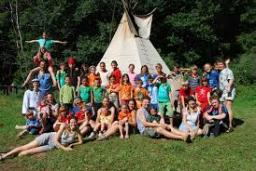Did you find an error or inaccuracy? Feel free to write us. Thank you!Tips to related online calculators
Do you have a linear equation or system of equations and looking for its solution? Or do you have a quadratic equation?
Do you want to convert length units?
Do you want to convert velocity (speed) units?
Do you want to convert time units like minutes to seconds?

## Related math problems and questions:

• Scouts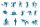Three scouts went on a three day trip. The second day went 4 km more than the first day. The third day went two times less than the first day. They went along 54 km. How many kilometers went every day together?
• Cyclist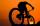Cyclist rode two-fifths of the way the first day. The second day drove 5 km less while traveled three-eighths of route. How many kilometers does he have to finish target?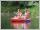Students of canoeists trip traveled in three days 102 km. The second day traveled 15% more than the first day and at the third day 9 km over the second day. How many kilometers are traveled each day?
• Tourists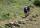From the cottage started first group of tourists at 7:00 AM at speed 4 km/h. The second started after them 21 minutes later at speed 6 km/h. How long and how many kilometers from the cottage will catch the first group?
• TruckThe truck passed 4550 km in 5 days. The first three days passed every day the same way. The fourth day passed 630 km and the fifth day was 920 km. How many km has passed the first three days?
• Train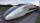A passenger train traveled for 2 hours 74 km. 3.1 hours after its departure started fast train and caught it on 186 km. How many km/h is different its average speeds?
• The touristThe tourist traveled 190km in 5 hours. Part of the journey passed at 5 km/h. The rest he went by bus at a speed of 60 km/h. How long has a bus going?
• Train from PragueThe first train from Prague started at 8:00 hour at 40 kilometers per hour. Train from Ostrava started at 9:20 at 80 km per hour. How many hours and how far from cities with trains meet if the distance of cities is 400 km.
• Train from BrnoFirst train started from Brno at 8 am at 60 km per hour. Second train started from Opava at 7 o'clock at the speed of 40 kilometers per hour. In how many hours and how far from cities trains meet when the distance of cities is 200 kilometers.
• Two trains meetFrom A started at 7:15 express train at speed 85 km/h to B. From B started passenger train at 8:30 in the direction to A and at speed 55 km/h. The distance A and B are 386 1/4 km. At what time and at what distance from B the two trains meet?
• Cycling trip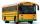At 8 am started a group of children from the camp to day cycling trip. After 9:00 weather deteriorated sharply and the leader decided to send for the children bus on the same route, which started at 10 hours. For how long and at what distance from the cam
• Aircrafts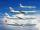Above the town hall tower flew the plane with constant speed 510 km/h and 15 minutes later the second plane at speed of 703 km/h. How long and how far from the town hall will be aircrafts caught up?
• Ascent and descent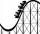Car goes 114 km track, which consists of ascent and descent at time of 1 hour 35 minutes. When climbing moves at the speed 48 km/h and downhill 25 m/s. What is the length of climb and descent?
• Forth and back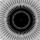Car drive from point A to point B at speed 78 km/h and back at 82 km/h. If went there and back at speed 81 km/h journey would take five minutes less. What is the distance between points A and B?
• Two cars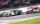Car A1 goes at average speed of 106 km/h and the second car A2 goes at 103 km/h. How many second will it take car A1 to circulate car A2? Assume that both cars are 5 meters long and the safety gap between cars is 1.5 meters.
• MotionFrom two different locations distant 232 km started against car and bus. The car started at 5:20 with average speed 64 km/h. Bus started at 7:30 with average speed 80 km/h. When they meet? How many kilometers went the bus?
• Car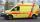The car drove some distance in 3 hours and 20 minutes. If it increases speed by 10 km/h, it goes this distance in 2.5 hours. Calculate the distance.